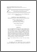# Pseudo τ - adic non adjacent form for scalar multiplication on Koblitz curves

## Citation

Yunos, Faridah and Mohd Atan, Kamel Ariffin and Kamel Ariffin, Muhammad Rezal and Md. Said, Mohamad Rushdan (2015) Pseudo τ - adic non adjacent form for scalar multiplication on Koblitz curves. Malaysian Journal of Mathematical Sciences, 9 (spec.). pp. 71-88. ISSN 1823-8343

## Abstract

In ECC, scalar multiplication is the dominant operation, namely computing nP from a point P on an elliptic curve where the multiplier n is an integer, defined as the point resulting from adding P+P+⋯+P, n times. The τ-NAF proposed by Solinas, is one of the most efficient algorithms to compute scalar multiplications on Koblitz curves. In this paper, we introduced an equivalent multiplier to τ-NAF namely pseudo TNAF. It is based on the idea of transforming the τ-NAF expression to a reduced τ-NAF that has been done by some researchers. It can eliminate the elliptic doublings in scalar multiplication method, and double the number of elliptic additions. We provide the formula for obtaining a total of lattice points in Voronoi region of modulo r+st where r+sτ an element of ring Z(τ). This helps us to find all the multipliers n that based on τ-NAF. We also discuss the estimation of operational costs when using pseudo TNAF as a multiplier of scalar multiplication.Preview
PDFView Item